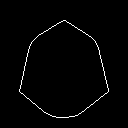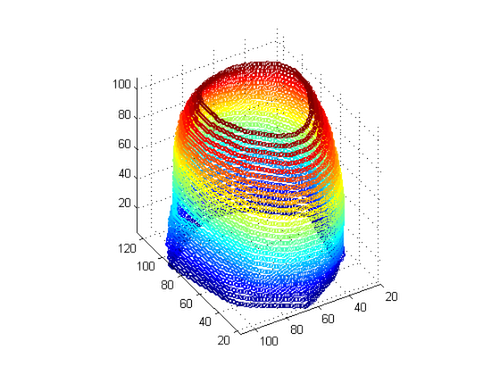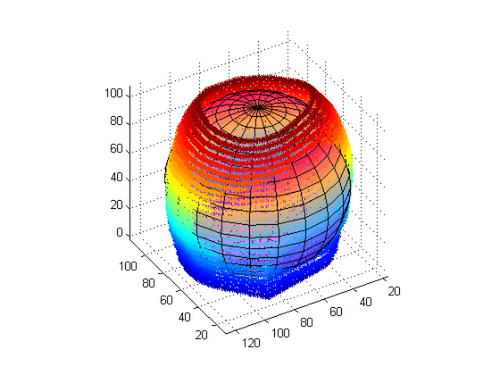# Fit a Sphere!

Sean's pick this week is Sphere Fit by Alan Jennings.

### Fitting a Sphere to Data

I recently had some data and wanted to fit a sphere through it so that I could find the radius of this sphere. As I started writing out an objective function for one of the Optimization Toolbox optimizers (yes I was taking the way too big a hammer approach), a quick query on the File Exchange brought up sphereFit, a pleasant discovery.

Let's see it in action:

% Load mri of a human head
D = squeeze(S.D);

% Generate data points on the edge of the head
for ii = size(D,3):-1:1;
% Perimeter of convex hull of each slice
M(:,:,ii) = bwperim(bwconvhull(D(:,:,ii)>50));
end% Find sub indices in our mask
idx = find(M); % find points in M
[rr,cc,pp] = ind2sub(size(M),idx); % sub indices
pp = pp.*floor(size(D,1)./size(D,3)); % rescale third dimension

% View data
figure;
scatter3(rr,cc,pp,10,pp);
daspect([1,1,1]);
view(-121,36);
axis tight;% Fit the Sphere:
fprintf(1,'\nRadius of sphere is %3.1f\nIt is centered at [%3.1f %3.1f %3.1f]\n',radius,cent);
Radius of sphere is 54.3
It is centered at [72.9 63.4 51.7]


Use Alan's example code to show the sphere through the points

scatter3(cc,rr,pp,25,pp,'*'); %points
hold on; % hold
daspect([1,1,1]); % equal axis so a sphere looks like a sphere
[Base_rr,Base_cc,Base_pp] = sphere(20);
axis tight;
view(-121,36);